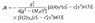# Calculate Alpha_s: Jet Multiplicity Rates

• serleon

#### serleon

Hi!
Any idea of how to calculate alpha_s (strong coupling constant) from jet multiplicities, that means using (1+1) and (2+1) jet events rates?
Thanks

Think about the Feynman diagrams which make up the processes. At leading order the 2 jet rate is has two quarks in the final state, while the 3 jet rate has 2 quarks and a gluon. The gluon is radiated from a quark line, so there will be an extra factor of alpha_s.

As I have found, the amplitude for e+e- --> q _q would be (see attach)...
Maybe any other resource or book where I could find the calculations for e+e- --> q _q g

#### Attachments

•2.JPG
11.9 KB · Views: 346